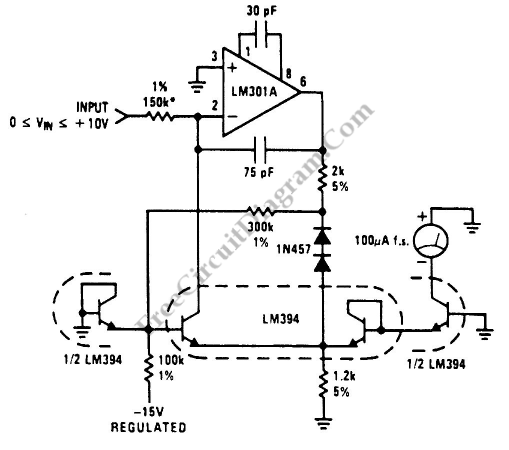# Accurate Low-Cost Square-Root ConverterThis is a Low-Cost Accurate Square-Root Circuit. This circuit is used to provide a square-root function with a good accuracy. The benefit of This circuit is cheap. This circuit produce a current output which can be used to be converted to voltage by a summing junction current-to-voltage converter or to drive a meter directly. This circuit requires 15V supply. The supply of this circuit should be stable because it is used as a reference. Here is the circuit:If the supply voltage is unstable, it will cause shift in output reading. For example the output current will be shifted 1/2% when 1% change in the 15V supply. This circuit does not require a positive supply because this circuit uses LM301A which its inputs can be used at the same voltage as the positive supply (ground). For temperature-compensate, this circuit uses 300KΩ and 1N457 diodes that will allow the the current through the diode-connected1/2 LM394. [Circuit’s schematic diagram source: seekic.com]